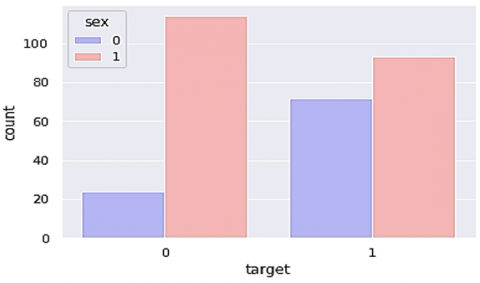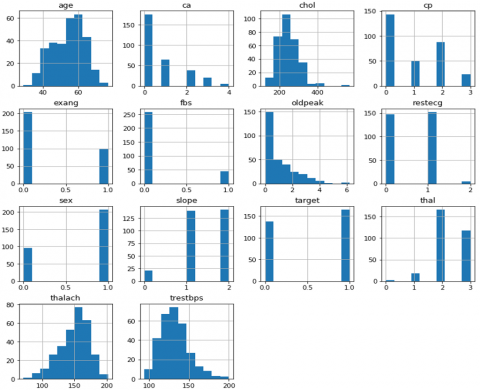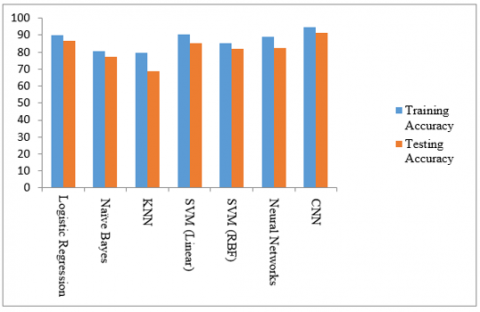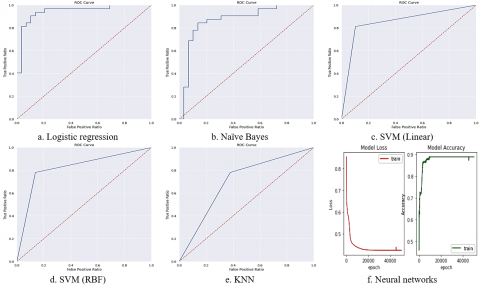# A Deep Learning Method for Prediction of Cardiovascular Disease Using Convolutional Neural Network

A Deep Learning Method for Prediction of Cardiovascular Disease Using Convolutional Neural Network
Vignan's Foundation for Science, Technology and Research deemed to be University, Vadlamudi, Guntur 522213, AP, India

Corresponding Author Email:
stk_cse@vignan.ac.in
Page:
601-606
|
DOI:
https://doi.org/10.18280/ria.340510
22 June 2020
|
Accepted:
3 October 2020
|
Published:
20 November 2020
| Citation

OPEN ACCESS

Abstract:

Heart disease is a very deadly disease. Worldwide, the majority of people are suffering from this problem. Many Machine Learning (ML) approaches are not sufficient to forecast the disease caused by the virus. Therefore, there is a need for one system that predicts disease efficiently. The Deep Learning approach predicts the disease caused by the blocked heart. This paper proposes a Convolutional Neural Network (CNN) to predict the disease at an early stage. This paper focuses on a comparison between the traditional approaches such as Logistic Regression, K-Nearest Neighbors (KNN), Naïve Bayes (NB), Support Vector Machine (SVM), Neural Networks (NN), and the proposed prediction model of CNN. The UCI machine learning repository dataset for experimentation and Cardiovascular Disease (CVD) predictions with 94% accuracy.

Keywords:

cardiovascular disease, heart attack, convolutional neural network, SVM, KNN, logistic regression, naïve Bayes, deep learning

1. Introduction

As per the latest survey by the WHO, 17.9 M people pass away every year. There is no surprise that by the year 2030 it will increase to 75 million. American Heart Association considers approximately every 40 seconds, and an American will have a heart attack. Many cardiovascular diseases (CVD) exist to kill humans by using detectable hazards such as tobacco usages, unwanted eating habits, physical dormancy, and deadly usage of liquor in a range of contexts. Human beings who are having CVD are at high risk of cardiac. The disease requires early detection and guidance on the use of short medications, as set out below. Overall, CVD comes to an end with fatty stores' production within the ducts and blood groups' output. It can also be linked to an injury to tissues, such as the head, eyes, heart, and kidneys. CVD is a major leading cause of death and injury in the United Kingdom  but can be stopped daily to a wide degree by maintaining a good lifestyle. Cardiac cases and strokes are usually caused by powerful events and are mostly caused by a clot that prevents blood flow to the mind or heart. The most commonly known aim behind this is the creation of the most inward-looking greasy shops.

This issue had created a lot of seriousness between researchers; one of the critical tasks in this is to predict the disease present in the human body. Even doctors are also not efficient in predicting the disease . However, they need a support system to predict the disease. Some of the algorithms are supported but need to improvise the system's performance beyond the existing system. Therefore, to help medicos, there is enormous research scope in predicting CVD disease in humans as support of medicos.

The Machine Learning algorithms are That type of support system proposed in this paper with deep learning technique. One of the deep learning algorithms, i.e., convolutional network (CNN) diagnose disease better than the existing methods. This CNN based model deals with a high volume of data. The advantage of CNN is everything is to be done by the network, such as preprocessing, feature extraction, prediction. The system accepts raw data.

The rest of the manuscript structured as follows: Section-2 describes the existing literature work. Section-3 explains the background of the machine learning algorithms and their functionalities. Section-4 gives an overview of the proposed methodology and also discussed the experimental results. Finally, conclusions in Section-5.

2. Related Work

Beunza et al.  studied the clinical machine learning approaches regarding their validity and accuracy, logistic regression, Random Forest, decision tree, SVM, and NN. Zhao et al.  carefully studied the cardiovascular break-down rate with pulse transition by time analysis, ML, and CNN models used for examination. The support vector machine outperformed each of the classification algorithms in the assessment of cardiovascular recognition. Chen et al.  expected to calculate one year of cardiovascular events in patients with severe DCM using ML models. The ML algorithm's contribution was 32 highlights from clinical information, and Information Gain (IG) selected significant highlights that were exceptionally relevant to cardiovascular events. The study  explored the cardiovascular infections in humans on medications. Two ML techniques have been used and taken into account by Base of Fisiologiab Clinica; the other one was an American dataset supported regional diabetes and the Stomach Associated Association and the Vault of kidney diseases.

Awan et al.  expected the usage of the Artificial Neural Network for heart infections. The goal is to use machine learning and pattern matching strategies to fix heart disease. Gjoreski et al.  predicted the Continuous Cardiovascular Disruption Detection of Heart Rhythm utilizing ML classifiers' collection. These techniques used to predict include sampling, segmentation, selection of functions. Cardiovascular Disease Risk Prediction Approach using computerized machine learning has been given in the study . An ML built model derived by the usage of auto-prognosis and an algorithmic method that, as a consequence, selects and implements the researchers used the ML simulation pipelines. Another ML method for the accurate determination of coronary artery disease was introduced by Abdar et al. . The progression method, named as N2 Genetic Optimizer Agent, was introduced in this methodology. Such tests are violent and essentially the same as the most robust findings in the sector.

Tang et al.  provided the Continuous Arrhythmia heartbeat recognition algorithm. This method used in this treatment is the parallel delta Modulations and Rotated Linear SVM. Photonic crystal enhanced fluorescence visualization Cardiovascular disorder immunoassay biomarker test with machine learning investigation was conducted by Squire et al. . PCA , PLSR Regression algorithms, and advanced ML Algorithm strategies are used in this study. Coronary artery disease, which is machine-based learning, was discussed by Alizadehsani et al. ; datasets tested, analyzed the weights, implementation measurements, and ML are the essential approaches that have been shattered down in this method. Research  used machine learning classifiers. The anticipation of hepatitis investigation, random forest classifier outflanked each of the classification models examined. Sajja et al.  proposed a medical assistive support system to classify the malignant and benign from lung CT scan images.

3. Background Methods

3.1 Logistic regression

It is one of the supervised learning and is used to estimate the target object value's possibility. It is a tool to calculate the statistical values and make results on binary output. In the linear method, which is calculated by the equation:

y = b0(x) + b1    (1)

whereas logistic regression  used in Eq. (1). In this, the constant b1 bias and the slope b0 tell the curve's steepness.

Prob $=\frac{1}{1+e^{-(y)}}$    (2)

Here, y is the linear model's output trained with logistic regression produce value between zero and one.

3.2 Naive Bayes

In the Naïve Bayes network , all features are independent. When there is a change in one feature, it does not affect another. This is suitable for large datasets. The assumption from Conditional independence is that an attribute value is independent of the values, which are from other attribute values in a class. Bayes' Theorem  is based on probability theory:

$\operatorname{prob}(\mathrm{x} \mid \mathrm{y})=\frac{\operatorname{prob}(\mathrm{x}) \mathrm{prob}(\mathrm{y} \mid \mathrm{x})}{\operatorname{prob}(\mathrm{y})}$    (3)

where,

prob (x|y) - x happens given that y happens,

prob(y|x) - y happens given that x happens,

prob(x) - x is on its own,

prob(y) - y is on its own.

3.3 Support Vector Machine (SVM)

SVM  is used both for regression and classification tasks. The SVM model represents the data in the space described so that the examples in various categories are divided by a distance as large as possible. That divides sensitive information with the maximum separable space between them and is calculated so that many of the points belong to one group fall on the plane's one side. This paper used two types of SVM kernels they are

3.3.1 Linear kernel

The dot product is performed among two observations. The equation of the linear kernel is in Eq. (4).

kernel(s, si)=sum(s*si)    (4)

From Eq. (4), observe that the product between two vectors, let say s and si, is the sum of the input values pair's multiplication.

RBF is mainly used in SVM classification, which maps input space in new dimensional space. Eq. (5) explains as

Kernel(s,si)=exp(−gamma*sum(s−si2))    (5)

Generally, a gamma range between 0 to 1. However, the default value for gamma is 0.1.

3.4 KNN

K-Nearest Neighbor  is an anti-parametric method, which is used for regression and classification. It is essentially a grouping method, consider the distance between a point and the coordinates (x, y) and its neighbors. The distance between the Euclidean is in Eq. (6) and its neighbors are determined from the point and eventually located in the region nearest to its neighboring points.

distance $D(x, y)=\sqrt{\sum_{i=1}^{n}\left(x_{i}-y_{i}\right)^{2}}$    (6)

Here, x, y are two points in Euclidean 'n' space, $x_{i}, y_{i}$ are two vectors starting from the initial point, 'n' means n-dimensional space.

4. Proposed Methodology & Experimental Results

4.1 Data set

For the experimentation, we considered Cleveland Heart Disease Dataset  from the UCI ML repository. This database contains 14 features; those are a person's age, gender, chest pain, treetops, chol, fbs, restecg, thalch, exang, oldpeak, slope, ca, thal and target. These 14 features are measured on 303 instances of heart disease patients. The particulars of the dataset were placed in Table 1.

4.2 Methodology

4.2.1 Preprocessing

At the principal level stage, the dataset is first cleaned and processed using preprocessing techniques using panda's package. The counterplot of sex and target attributes group is shown in Figure 1.

After that, using the data visualization procedure, the data frame attributes are shown in Figure 2, as histograms.

## 1.pngFigure 1. Counter plot grouped by sex vs. target

Table 1. Cleveland dataset details

 Attribute Name Description Value Type Values Range Age in years Numerical sex Gender info Nominal 1 - m, 0 - f Chest pain Type of cp Nominal 1, 2, 3, 4 trestbps resting blood pressure Numerical chol cholestoral (mg/dl) Numerical fbs fasting blood sugar > (120 mg/dl) Nominal 1 = T; 0 = F restecg resting electrocardiographic Nominal 0, 1, 2 thalach max heart rate Numerical exang exercise Nominal 1 - Y, 0 - N oldpeak depression induced by exercise with respect to rest Numerical slope Peak exercise Nominal 1, 2, 3 ca # of major vessels Numerical thal t3 - normal, t6 - fixed defect, t7 - reversable defect Numerical Target Diagnosis of disease Numerical Between 0,1

## 2.pngFigure 2. Histograms of data frame attributes

4.2.2 Proposed work

The proposed convolutional architecture contains the input layer followed by a convolutional layer with 16 kernels along with activation function as ReLU, in the subsequent layer 25% of the nodes is dropped by dropout layer. Again, the convolutional layer was performed with eight kernels with previous parameters, also applied the dropout layer with 25%. For prediction probability calculations, added an output layer.

The proposed architecture is shown in Figure 3. The cleaned data is split into 80% training and 20% testing for training and testing purposes. The same dataset is tested with different machine learning classifiers such as Logistic Regression (LR), NB, KNN, and SVM with different kernels, such as linear and RBF and simple neural networks. In this paper, we proposed a CNN to predict the accuracy of whether a patient had a cardio disease or not. 89.91 training accuracy and 86.83% testing accuracy.

## 3.pngFigure 3. Proposed architecture

The proposed convolutional network model accuracy is compared with the existing ML models which were depicted in Table 2. Logistic regression achieved 89.91%. Naïve Bayes achieved 80.62% training accuracy and 77.04% testing accuracy. KNN achieved 79.76% training accuracy and 68.86% testing accuracy. SVM (Linear) achieved 90.61% training accuracy and 86.83% testing accuracy. SVM (RBF) achieved 85.43% training accuracy and 81.96% testing accuracy. Neural Network achieved 88.95% training accuracy and 86.97% testing accuracy. The proposed network achieved 95.04% training accuracy and 94.78% testing accuracy. The graphical representation of existing and proposed method accuracies is shown in Figure 4. Figure 5 represents the ROC curves of LR, NB, KNN, SVM (Linear), SVM (RBF), and Neural Networks.

Table 2. Accuracies of different methods on Cleveland dataset

 Model Training Accuracy Testing Accuracy Logistic Regression 89.91 86.83 Naive Bayes 80.62 77.04 KNN 79.76 68.86 SVM Linear 90.61 85.29 RBF 85.43 81.96 Neural Network 88.95 86.97 Proposed Network 95.04 94.78

## 4.pngFigure 4. Graphical representation of accuracies in different models

## 5.pngFigure 5. ROC curves

5. Conclusion

Many of the state-of-the-art algorithms are not suitable to predict CVD disease correctly. Even doctors are also unable to predict the disease accurately. So, the proposed system supports medicos for prediction. In this script, we proposed a convolutional neural network-based model to predict the disease. And also, the paper gave a comparison between proposed work and state-of-the-art algorithms. The proposed model consists of two convolutional layers, two dropout layers, and an output layer. The reported accuracy by this model is 94.78% to predict disease on the UCI-ML Cleveland dataset. The proposed network deals with a large volume of data. Another advantage of this model is preprocessing, feature extraction and prediction done by the model itself, whereas the existing algorithm used different methods for each task.

References

 Bhatnagar, P., Wickramasinghe, K., Wilkins, E., Townsend, N. (2016). Trends in the epidemiology of cardiovascular disease in the UK. Heart, 102(24): 1945-1952. https://doi.org/10.1136/heartjnl-2016-309573

 Jabbar, M.A., Chandra, P., Deekshatulu, B.L. (2012). Prediction of risk score for heart disease using associative classification and hybrid feature subset selection. 2012 12th International Conference on Intelligent Systems Design and Applications (ISDA), Kochi, pp. 628-634. https://doi.org/10.1109/ISDA.2012.6416610

 Beunza, J.J., Puertas, E., García-Ovejero, E., Villalba, G., Condes, E., Koleva, G., Hurtado, C., Landecho, M.F. (2019). Comparison of machine learning algorithms for clinical event prediction (risk of coronary heart disease). Journal of Biomedical Informatics, 97: 103257. https://doi.org/10.1016/j.jbi.2019.103257

 Zhao, L., Liu, C., Wei, S., Liu, C., Li, J. (2019). Enhancing detection accuracy for clinical heart failure utilizing pulse transit time variability and machine learning. IEEE Access, 7: 17716-17724. https://doi.org/10.1109/ACCESS.2019.2895230

 Chen, R., Lu, A., Wang, J., Ma, X., Zhao, L., Wu, W., Du, Z., Fei, H., Lin, Q., Yu, Z., Liu, H. (2019). Using machine learning to predict one-year cardiovascular events in patients with severe dilated cardiomyopathy. European Journal of Radiology, 117: 178-183. https://doi.org/10.1016/j.ejrad.2019.06.004

 Mezzatesta, S., Torino, C., De Meo, P., Fiumara, G., Vilasi, A. (2019). A machine learning-based approach for predicting the outbreak of cardiovascular diseases in patients on dialysis. Computer Methods and Programs in Biomedicine, 177: 9-15.

 Awan, S.M., Riaz, M.U., Khan, A.G. (2018). Prediction of heart disease using artificial neural network. VFAST Transactions on Software Engineering, 13(3): 102-112. http://dx.doi.org/10.21015/vtse.v13i3.511

 Gjoreski, M., Simjanoska, M., Gradišek, A., Peterlin, A., Gams, M., Poglajen, G. (2017). Chronic heart failure detection from heart sounds using a stack of machine-learning classifiers. 2017 International Conference on Intelligent Environments (IE), Seoul, pp. 14-19. https://doi.org/10.1109/IE.2017.19

 Alaa, A.M., Bolton, T., Di Angelantonio, E., Rudd, J.H., van Der Schaar, M. (2019). Cardiovascular disease risk prediction using automated machine learning: A prospective study of 423,604 UK Biobank participants. PloS One, 14(5): e0213653. https://doi.org/10.1371/journal.pone.0213653

 Abdar, M., Książek, W., Acharya, U.R., Tan, R.S., Makarenkov, V., Pławiak, P. (2019). A new machine learning technique for an accurate diagnosis of coronary artery disease. Computer Methods and Programs in Biomedicine, 179: 104992. https://doi.org/10.1016/j.cmpb.2019.104992

 Tang, X., Ma, Z., Hu, Q., Tang, W. (2019). A real-time arrhythmia heartbeats classification algorithm using parallel delta modulations and rotated linear-kernel support vector machines. IEEE Transactions on Biomedical Engineering, 67(4): 978-986. https://doi.org/10.1109/TBME.2019.2926104

 Squire, K.J., Zhao, Y., Tan, A., Sivashanmugan, K., Kraai, J.A., Rorrer, G.L., Wang, A.X. (2019). Photonic crystal-enhanced fluorescence imaging immunoassay for cardiovascular disease biomarker screening with machine learning analysis. Sensors and Actuators B: Chemical, 290: 118-124. https://doi.org/10.1016/j.snb.2019.03.102

 Kalluri, H.K., Prasad, M.V., Agarwal, A. (2012). Palmprint identification based on wide principal lines. Proceedings of the International Conference on Advances in Computing, Communications and Informatics, pp. 918-924. https://doi.org/10.1145/2345396.2345544

 Alizadehsani, R., Abdar, M., Roshanzamir, M., Khosravi, A., Kebria, P.M., Khozeimeh, F., Acharya, U.R. (2019). Machine learning-based coronary artery disease diagnosis: A comprehensive review. Computers in Biology and Medicine, 111: 103346. https://doi.org/10.1016/j.compbiomed.2019.103346

 Kumar, N.K., Vigneswari, D. (2019). Hepatitis-infectious disease prediction using classification algorithms. Research Journal of Pharmacy and Technology, 12(8): 3720-3725. https://doi.org/10.5958/0974-360X.2019.00636.X

 Sajja, T.K., Devarapalli, R.M., Kalluri, H.K. (2019). Lung cancer detection based on CT scan images by using deep transfer learning. Traitement du Signal, 36(4): 339-344. https://doi.org/10.18280/ts.360406

 Balugani, E., Lolli, F., Butturi, M.A., Ishizaka, A., Sellitto, M.A. (2020). Logistic regression for criteria weight elicitation in PROMETHEE-based ranking methods. In: Ahram T., Karwowski W., Vergnano A., Leali F., Taiar R. (eds) Intelligent Human Systems Integration 2020. Advances in Intelligent Systems and Computing, vol 1131. Springer, Cham. https://doi.org/10.1007/978-3-030-39512-4_74

 Kaur, G., Oberoi, A. (2020). Novel approach for brain tumor detection based on Naïve Bayes classification. In: Sharma N., Chakrabarti A., Balas V. (eds) Data Management, Analytics and Innovation. Advances in Intelligent Systems and Computing, vol 1042. Springer, Singapore. https://doi.org/10.1007/978-981-32-9949-8_31

 Land, W.H., Schaffer, J.D. (2020). The support vector machine. In the Art and Science of Machine Intelligence, Springer, Cham, pp. 45-76. https://doi.org/10.1007/978-3-030-18496-4_2

 Tang, H., Xu, Y., Lin, A., Heidari, A.A., Wang, M., Chen, H., Luo, Y., Li, C. (2020). Predicting green consumption behaviors of students using efficient firefly grey wolf-assisted K-nearest neighbor classifiers. IEEE Access, 8: 35546-35562. https://doi.org/10.1109/ACCESS.2020.2973763

 Christopher, B. (2016). Replication Data for: Cleveland Heart Disease. https://doi.org/10.7910/DVN/QWXVNT, Harvard Dataverse, V1, UNF:6:uUXnE2XOKvaGcPfH8fzDpw== [fileUNF], accessed on 26 February 2020.